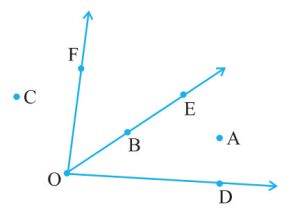## Ex 4.3 Question 1.

Name the angles in the given figure.Solution:

There are four angles = ∠DAB, ∠ABC, ∠BCD, and ∠CDA

## Ex 4.3 Question 2.

In the given diagram, name the points(s)

(a) In the interior of ∠DOE

(b) In the exterior of ∠EOF

(c) On ∠EOFSolution:

(a) The point in the interior of ∠DOE = A.

(b) The point in the exterior of ∠EOF = C, A, and D.

(c) The points on ∠EOF = E, B, O, and F.

## Ex 4.3 Question 3.

Draw rough diagrams of two angles such that they have

(a) One point in common

(b) Two points in common

(c) Three points in common

(d) Four points in common

(e) One ray in common

Solution:error: Content is protected !!